Warning

This documents an unmaintained version of NetworkX. Please upgrade to a maintained version and see the current NetworkX documentation.

# degree_centrality¶

degree_centrality(G, nodes)[source]

Compute the degree centrality for nodes in a bipartite network.

The degree centrality for a node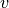is the fraction of nodes connected to it.

Parameters: G (graph) – A bipartite network nodes (list or container) – Container with all nodes in one bipartite node set. centrality – Dictionary keyed by node with bipartite degree centrality as the value. dictionary

betweenness_centrality(), closeness_centrality(), sets(), is_bipartite()

Notes

The nodes input parameter must conatin all nodes in one bipartite node set, but the dictionary returned contains all nodes from both bipartite node sets.

For unipartite networks, the degree centrality values are normalized by dividing by the maximum possible degree (which is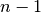where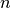is the number of nodes in G).

In the bipartite case, the maximum possible degree of a node in a bipartite node set is the number of nodes in the opposite node set . The degree centrality for a nodein the bipartite sets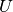withnodes and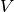with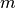nodes is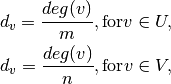where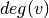is the degree of node.

References

  Borgatti, S.P. and Halgin, D. In press. “Analyzing Affiliation Networks”. In Carrington, P. and Scott, J. (eds) The Sage Handbook of Social Network Analysis. Sage Publications. http://www.steveborgatti.com/papers/bhaffiliations.pdf